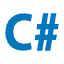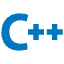TO

# Usage instructions :

Enter your question or code in the input box below, then click the button you want to execute.

## For example input: sum code in java;

Here's a simple Java program that calculates and prints the sum:

``````int sum = 0;
for (int i = 1; i <= 10; i++) {
sum += i;
}
System.out.println("The sum is: " + sum);``````

## Languages Supported

Convert code across 49+ programming languages### Android Code Converter### Angular Code Converter### Arduino Code Converter### Bash Code Converter### Binary Code Code Converter### C Code Converter### C# Code Converter### C++ Code Converter### CSS Code Converter### Django Code Converter### Docker Code Converter### Esp 32 Code Converter### Esp 8266 Code Converter### Go Code Converter### Groovy Code Converter### Hex Code Code Converter### HTML Code Converter### HTML JS CSS Code Converter### Java Code Converter### JavaScript Code Converter### Kotlin Code Converter### Kubernetes Code Converter